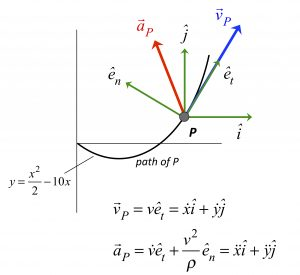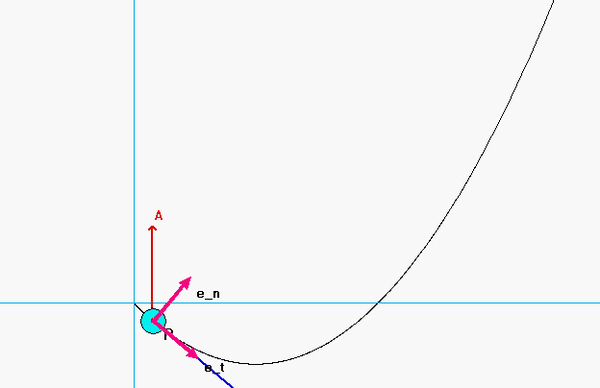# Joint kinematic descriptionParticle P moves on a path described as above in terms of Cartesian components. The x-component of velocity for P is known to be a constant. What we want, however, is an understanding of the path description; in particular, what is the rate of change of speed of P as it moves along the path?

To do this, we first first the velocity and acceleration in terms of its xy-components. Since the x-component of acceleration is zero, the acceleration of P must be solely in the y-direction. Then, from the above path kinematics equation for acceleration, we know that the rate of change of speed is simply the projection of the acceleration onto the direction tangent to the path.The animation above shows the acceleration to be strictly in the y-direction, as expected. The rate of change of speed is the projection of this acceleration vector onto the unit tangent direction.

• We see that for the first portion of this path when P is moving downward, the speed is decreasing since the projection of the acceleration is negative.
• For the second portion of the path when P is moving upward, the speed is increasing since the projection of the acceleration is positive.